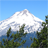# In Survey 123 Connect is there a way to format the number values as currency? I can concatenate the \$ in front of the numbers, but not sure how to get the commas in.

681
1
Jump to solution
12-18-2018 07:55 AMNew Contributor II

Looking for a solution in Survey 123 Connect.

1 Solution

Accepted Solutionsby
Occasional Contributor II

I don't think there's a built-in way to do this. This is discussed in this thread:

How to format display of calculated result in Survey123

I have done this using a combination of string-length(), concat(), and substr(), however. I broke the number into sub-strings based on its length using "if" statements, and concatenated commas between the sub-strings. It's not clean, but it has worked for me.

Due to the hard-coding of sub-strings, this doesn't work with negative numbers (though it could if you made some modifications).

An example:

typenamelabelconstraintcalculationbind::esri:fieldTypebind::type
integernumNumber.>=0
calculatenum_lenLength of numberstring-length(\${num})nullint
calculatenum_w_commasNumber with commasif(\${num_len}=4, concat(substr(\${num},0,1),",",substr(\${num},1,4)), if(\${num_len}=5, concat(substr(\${num},0,2),",",substr(\${num},2,5)), if(\${num_len}=6, concat(substr(\${num},0,3),",",substr(\${num},3,6)), if(\${num_len}=7, concat(substr(\${num},0,1),",",substr(\${num},1,4),",",substr(\${num},4,7)), if(\${num_len}=8, concat(substr(\${num},0,2),",",substr(\${num},2,5),",",substr(\${num},5,8)), if(\${num_len}=9, concat(substr(\${num},0,3),",",substr(\${num},3,6),",",substr(\${num},6,9)),\${num}))))))nullstring
textformatted_numberFormatted numberconcat("\$",\${num_w_commas})

Attached are a few examples of integer inputs and the formatted text calculated.

1 Replyby
Occasional Contributor II

I don't think there's a built-in way to do this. This is discussed in this thread:

How to format display of calculated result in Survey123

I have done this using a combination of string-length(), concat(), and substr(), however. I broke the number into sub-strings based on its length using "if" statements, and concatenated commas between the sub-strings. It's not clean, but it has worked for me.

Due to the hard-coding of sub-strings, this doesn't work with negative numbers (though it could if you made some modifications).

An example:

typenamelabelconstraintcalculationbind::esri:fieldTypebind::type
integernumNumber.>=0
calculatenum_lenLength of numberstring-length(\${num})nullint
calculatenum_w_commasNumber with commasif(\${num_len}=4, concat(substr(\${num},0,1),",",substr(\${num},1,4)), if(\${num_len}=5, concat(substr(\${num},0,2),",",substr(\${num},2,5)), if(\${num_len}=6, concat(substr(\${num},0,3),",",substr(\${num},3,6)), if(\${num_len}=7, concat(substr(\${num},0,1),",",substr(\${num},1,4),",",substr(\${num},4,7)), if(\${num_len}=8, concat(substr(\${num},0,2),",",substr(\${num},2,5),",",substr(\${num},5,8)), if(\${num_len}=9, concat(substr(\${num},0,3),",",substr(\${num},3,6),",",substr(\${num},6,9)),\${num}))))))nullstring
textformatted_numberFormatted numberconcat("\$",\${num_w_commas})

Attached are a few examples of integer inputs and the formatted text calculated.